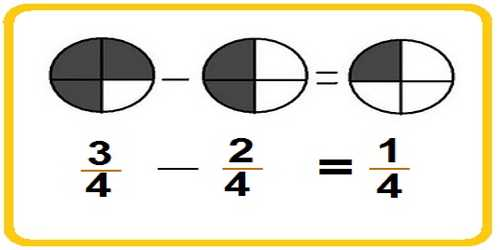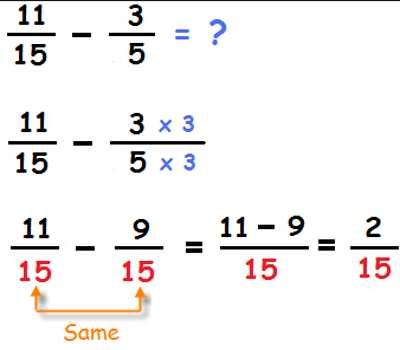Mathematic

# Subtracting Fractions with DenominatorSubtracting Fractions with Denominator

Subtracting fractions is done differently than the usual numbers. The denominator is the part of a fraction that is below the line and that functions as the divisor of the numerator.

It’s easy to add and subtract like fractions, or fractions with the same denominator. You just add or subtract the numerators and keep the same denominator. The tricky part comes when you add or subtract fractions that have different denominators. To do this, you need to know how to find the least common denominator. In an earlier lesson, you learned how to simplify, or reduce, a fraction by finding an equivalent, or equal, fraction where the numerator and denominator have no common factors. To do this, you divided the numerator and denominator by their greatest common factor.Subtracting Fractions with the Same Denominator

Fractions consist of two numbers. The top number is called the numerator. The bottom number is called the denominator.

numerator  / denominator

To subtract two fractions with the same denominator, subtract the numerators and place that difference over the common denominator.

Subtracting Fractions with Different Denominators

• To Subtract Fractions with different denominators:
• Find the Lowest Common Denominator (LCD) of the fractions
• Rename the fractions to have the LCD
• Subtract the numerators of the fractions
• The difference will be the numerator and the LCD will be the denominator of the answer.
• Simplify the Fraction

Example: Find the difference between 3/12 and 2/9.

• Determine the Greatest Common Factor of 12 and 9 which is 3
• Either multiply the numbers and divide by the GCF (9*12=108, 108/3=36)
• OR – Divide one of the numbers by the GCF and multiply the answer times the other number (12/3=4, 9*4=36)
• Rename the fractions to use the Lowest Common Denominator (3/12=9/36, 2/9=8/36)
• The result is 9/36 – 8/36
• Subtract the numerators and put the difference over the LCD = 1/36
• Simplify the fraction if possible. In this case, it is not possible.

Information Source: